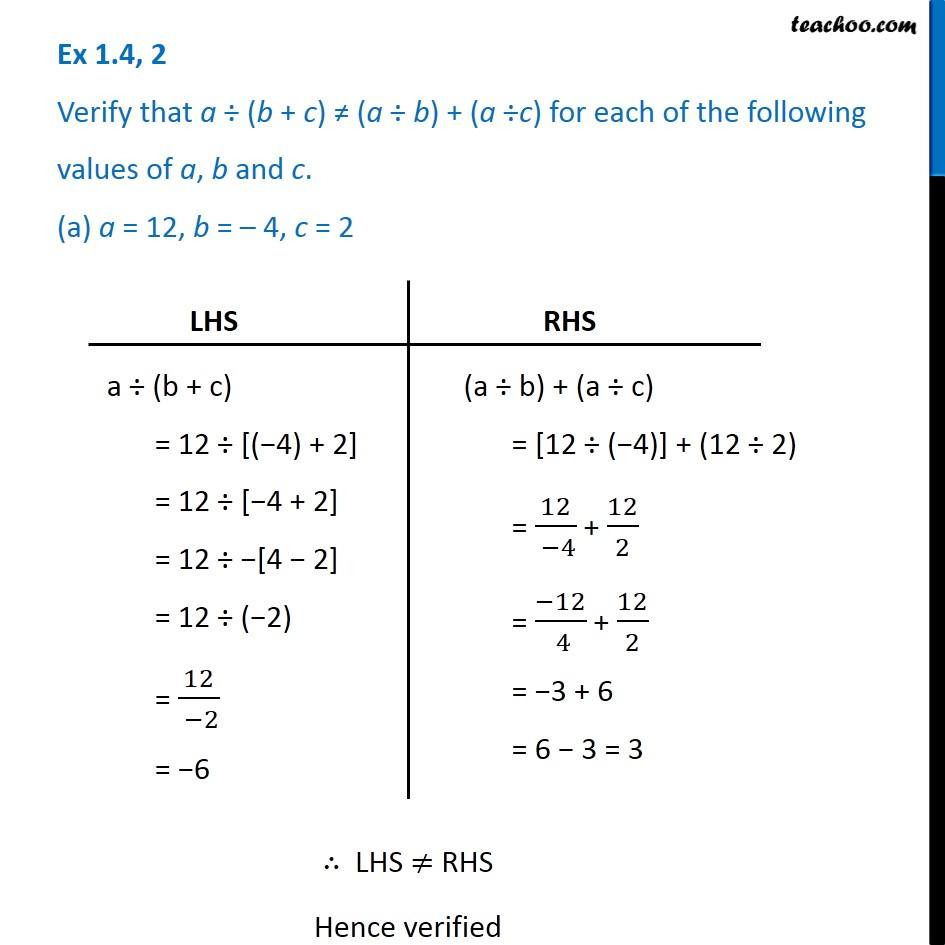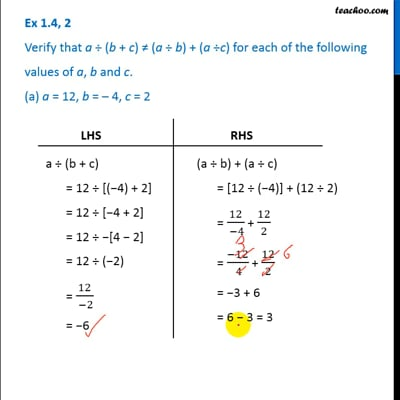Ex 1.4

Chapter 1 Class 7 Integers
Serial order wiseThis video is only available for Teachoo black users

### Transcript

Ex 1.4, 2 Verify that a (b + c) (a b) + (a c) for each of the following values of a, b and c. (a) a = 12, b = 4, c = 2 LHS a (b + c) = 12 [( 4) + 2] = 12 [ 4 + 2] = 12 [4 2] = 12 ( 2) = 12/( 2) = 6 RHS (a b) + (a c) = [12 ( 4)] + (12 2) = 12/( 4) + 12/2 = ( 12)/( 4) + 12/2 = 3 + 6 = 6 3 = 3 LHS RHS Hence verified